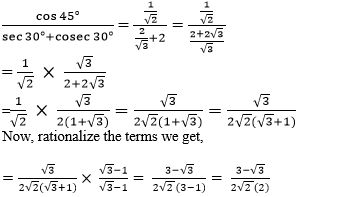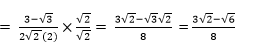Newbie

# Evaluate the following: (iii) cos 45°/(sec 30°+cosec 30°) Q.1(3)

• 0

Today i am solving the introduction of trigonometry of class 10 ncert . How i solve the question of exercise 8.2 question no.1(3) . Give me the best and easy solution of this question.Evaluate the following: (iii) cos 45°/(sec 30°+cosec 30°)

Share

1. cos 45°/(sec 30°+cosec 30°)

We know that,

cos 45° = 1/√2

sec 30° = 2/√3

cosec 30° = 2

Substitute the values, we getNow, multiply both the numerator and denominator by √2 , we getTherefore, cos 45°/(sec 30°+cosec 30°) = (3√2 – √6)/8

• 0